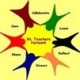## Parents

As parents you will have quite a few questions about Mathematical literacy, if your child should take it and so forth. We will use this page to collect some resources for you to understand what an amazing subject Mathematical literacy is and why your child SHOULD TAKE IT!

What is mathematical literacy?

Mathematical Literacy provides learners with an awareness and understanding of the role that mathematics has in the modern world. Mathematical Literacy is a subject driven by life-related applications of mathematics. It enables learners to develop the ability and confidence to think numerically and spatially in order to interpret and critically analyse everyday situations and solve problems.”
Source: The LEARNING PROGRAMME GUIDELINES for Mathematical Literacy, National Curriculum Statement for Grades 10 – 12 (General), 29 April 2005 – page 7

_____________________________________________________

What is the purpose of mathematical literacy?

he inclusion of Mathematical Literacy as a fundamental subject in the FET curriculum will ensure that our citizens of the future are highly numerate consumers of mathematics. In the teaching and learning of Mathematical Literacy learners will be provided with opportunities to engage with real life problems in different contexts (i.e. real life situations) and so consolidate and extend basic mathematical skills. Mathematical literacy will thus result in the ability to understand mathematical terminology and make sense of numerical and spatial information communicated in tables, graphs, diagrams and texts. Mathematical Literacy will, furthermore, develop the use of basic mathematical skills in critically analysing situations and creatively solving everyday problems.
Source: The LEARNING PROGRAMME GUIDELINES for Mathematical Literacy, National Curriculum Statement for Grades 10 – 12 (General), 29 April 2005 – page 7)

_____________________________________________________

What will mathematical literacy learners learn?

Learners of Mathematical Literacy will learn how to:

• Use a basic calculator.
• Perform basic arithmetical operations.
• Work with relationships between arithmetical operations.
• Work with simple formulae, including formulae for perimeter; area and volume; and speed and time.
• Estimate and check estimates against the situation.
• Work with and apply the concepts of ratio/proportion, percentage and rate.
• Determine input and output values for formulae (solve equations).
• Determine and plot the points for different graphs.
• Interpret information and trends communicated through graphs.
• Measure lengths, distances, volumes and mass (weight).
• Convert between units of measurement.
• Draw and interpret scale drawings.
• Use grids, scales and maps.
• Collect information to answer questions.
• Organise data using tallies and tables.
• Summarise data using the measures of mean, median and mode.
• Represent data using various data graphs, including pie charts, histograms and bar graphs.
• List the possible outcomes of an event.
• Estimate the likelihood of different outcomes.
• Critique interpretations of data.
• Formulate questions.
• Round up, round down and round off.
• Describe trends.
• Work with linear and constant relationships.
• Distinguish between piecewise and continuous relationships.
• Read and interpret information presented in tables.
• Anticipate the impact of interest.

Source: NATIONAL CURRICULUM STATEMENT GRADES 10-12 TEACHER GUIDE MATHEMATICAL LITERACY January 2006 Page 5

________________________________________________

Why do they need to know all of the above?

So that they are able to:

• Plan personal finances including
• understanding income and expenditure to plan a basic budget
• recognising the impact of interest rates.
• Calculate profit margins, loss and breakeven points in simple transactions.
• Plan for the repayment of a loan and anticipate associated bank costs.
• Convert between currencies.
• Plan and schedule events to meet deadlines and demands.
• Make sense of utility costs such as water, electricity, sewerage and rates.
• Choose between different options based on their value for money.
• Critique articles and advertisements in the media based on data and illustrated through graphs.
• Make lifestyle choices, such as the food they should eat in relation to the energy they use in their day-to-day lives.
• Calculate and interpret health indicators, such as Body Mass index (BMI).
• Sort and classify items according to criteria.
• Read maps to plan trips.
• Read and develop plans for simple structures.
• Understand the role and purpose of the gears on a bicycle.
• Calculate the time it takes to complete a journey.
• Anticipate which seats in the stadium will give the best view of the game.
• Predict all the possible outcomes of a sports tournament and anticipate the most likely winner.
• Understand that games of chance have no patterns.
• Develop arguments based on facts and the interpretations of facts.
• Use resources in economical and responsible ways.

Source: NATIONAL CURRICULUM STATEMENT GRADES 10-12 TEACHER GUIDE MATHEMATICAL LITERACY January 2006 Page 5

_____________________________________________________

What is the difference between maths and maths literacy?

• Maths Literacy focuses on the role of mathematics in the real world, whereas Mathematics focuses on the discipline of mathematics.
• With maths literacy, relevant current contexts are used, whereas with maths, applications are important, but do not have to be only real life contexts.
• With ML only basic mathematics is needed and a few new concepts are introduced in Grades 10 and 11. In Maths, content is expanded on as the learners progress from one year to another.
• In ML the contexts become more complex from year to year whereas in maths both the content and contexts become more complex and advanced

Source: Mathematical literacy workshop notes (Radmaste)

______________________________________________________

Is ml an easy option?
NO. Mathematical literacy is a different kind of mathematics, not a different, lower level of mathematics. Mathematical literacy will be at least as demanding as mathematics to teach and certainly as challenging for pupils to learn.
1.February Newsletter « Maths literacy teachers’ blog Says:
2.Mathsliteracy Resources (weekly) « Maths literacy teachers’ blog Says: